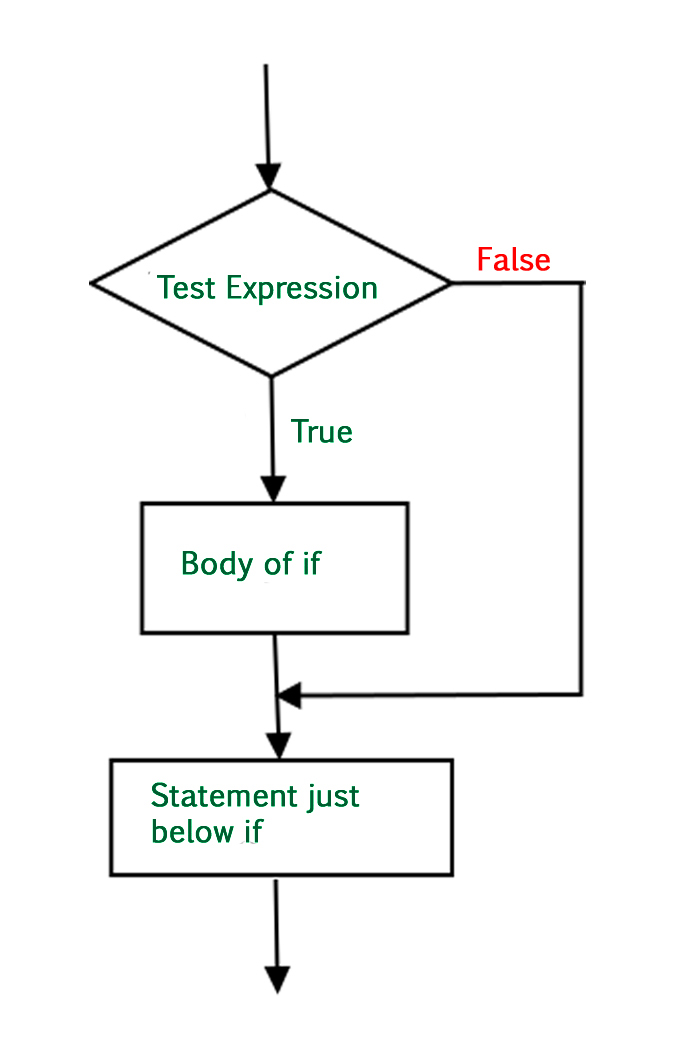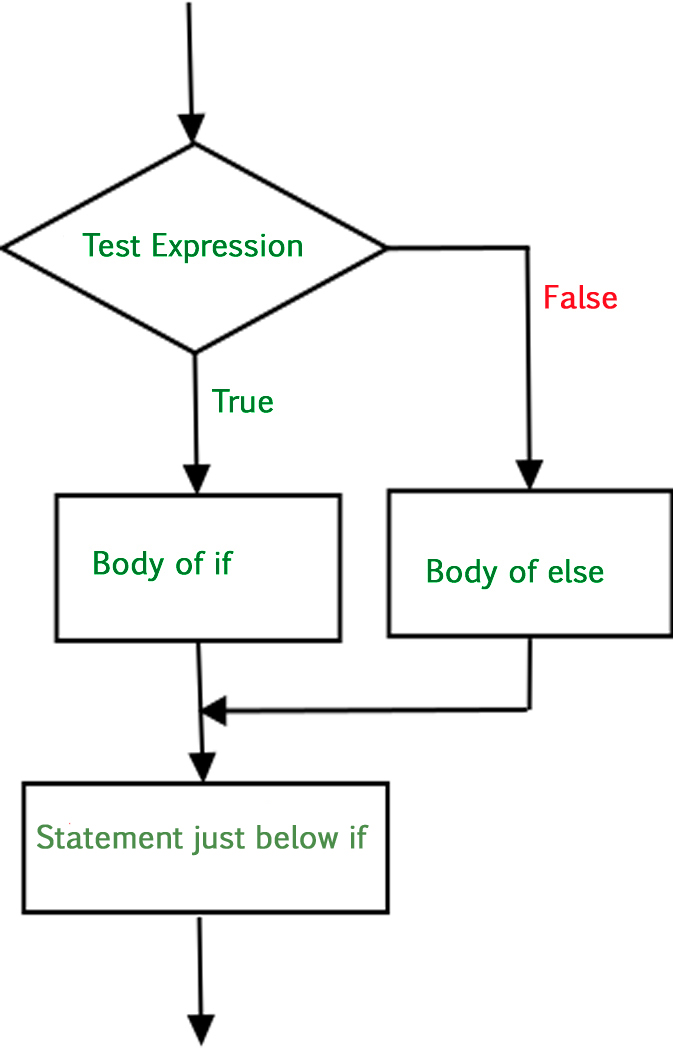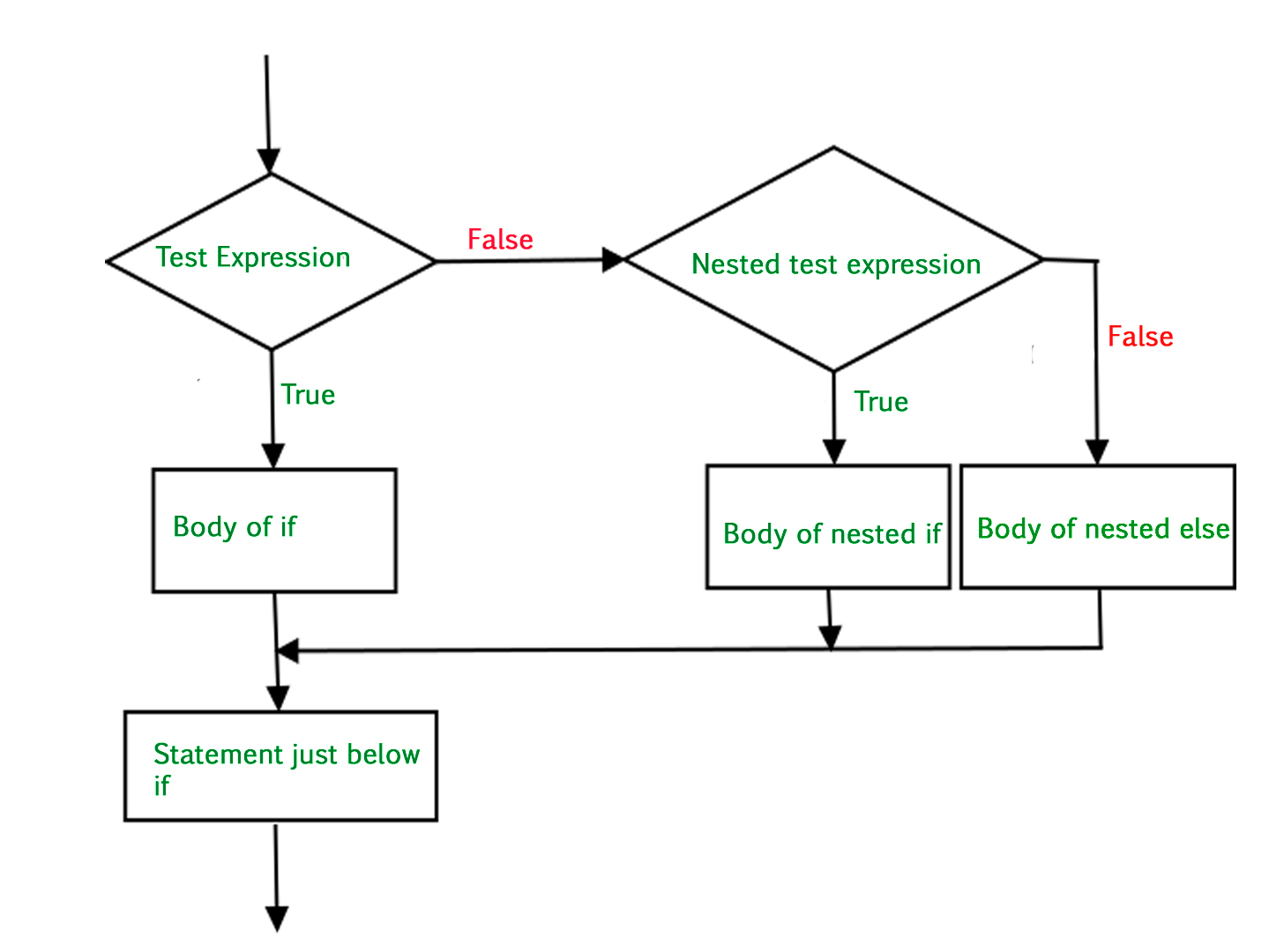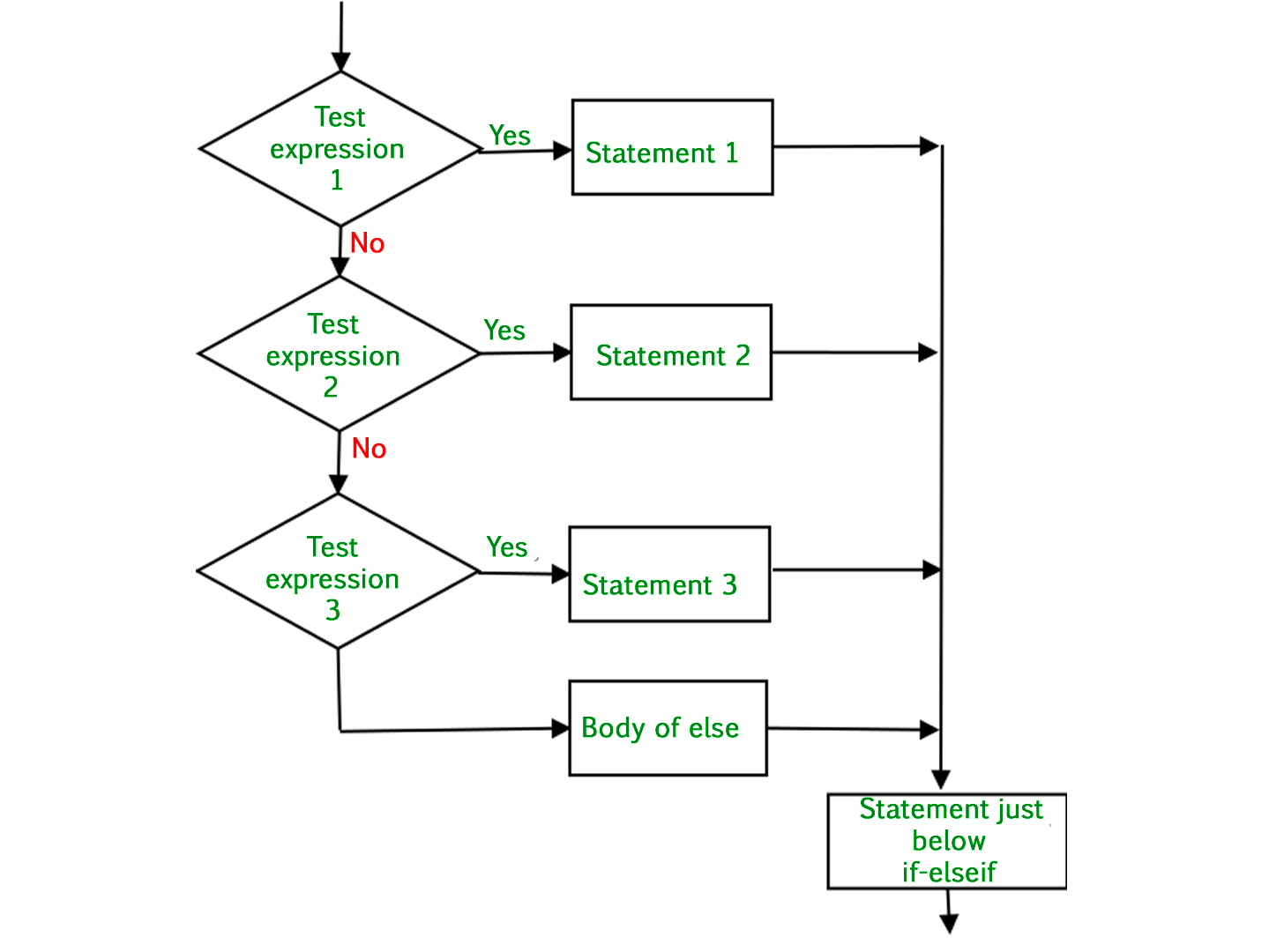Related Articles
Python3 – if , if..else, Nested if, if-elif statements
• Last Updated : 10 May, 2020

There come situations in real life when we need to do some specific task and based on some specific conditions and, we decide what should we do next. Similarly there comes a situation in programming where a specific task is to be performed if a specific condition is `True`. In such cases, conditional statements can be used. The following are the conditional statements provided by Python.

Let us go through all of them.

## if Statement

If the simple code of block is to be performed if the condition holds true than if statement is used. Here the condition mentioned holds true then the code of block runs otherwise not.

Syntax:

```if condition:
# Statements to execute if
# condition is true```

Flowchart:-Example:

 `# if statement example``if` `10` `> ``5``:``   ``print``(``"10 greater than 5"``)`` ` `print``(``"Program ended"``)`

Output:

```10 greater than 5
Program ended
```

Indentation(White space) is used to delimit the block of code. As shown in the above example it is mandatory to use indentation in Python3 coding.

## if..else Statment

In conditional if Statement the additional block of code is merged as else statement which is performed when if condition is false.

Syntax:

```if (condition):
# Executes this block if
# condition is true
else:
# Executes this block if
# condition is false
```

Flow Chart:-Example 1:

 `# if..else statement example``x ``=` `3``if` `x ``=``=` `4``:``   ``print``(``"Yes"``)``else``:``   ``print``(``"No"``)`

Output:

```No
```

Example 2: You can also chain if..else statement with more than one condition.

 `# if..else chain statement ``letter ``=` `"A"`` ` `if` `letter ``=``=` `"B"``:``  ``print``(``"letter is B"``)``   ` `else``: ``     ` `  ``if` `letter ``=``=` `"C"``:``    ``print``(``"letter is C"``)``     ` `  ``else``:``       ` `    ``if` `letter ``=``=` `"A"``:``      ``print``(``"letter is A"``)``       ` `    ``else``: ``      ``print``(``"letter isn't A, B and C"``)`

Output:

```letter is A
```

## Nested if Statement

if statement can also be checked inside other if statement. This conditional statement is called nested if statement. This means that inner if condition will be checked only if outer if condition is true and by this, we can see multiple conditions to be satisfied.

Syntax:

```if (condition1):
# Executes when condition1 is true
if (condition2):
# Executes when condition2 is true
# if Block is end here
# if Block is end here
```

Flow chart:-Example:

 `# Nested if statement example``num ``=` `10`` ` `if` `num > ``5``:``   ``print``(``"Bigger than 5"``)`` ` `   ``if` `num <``=` `15``:``      ``print``(``"Between 5 and 15"``)`

Output:

```Bigger than 5
Between 5 and 15
```

## if-elif Statment

The if-elif statement is shoutcut of if..else chain.While using if-elif statement at the end else block is added which is performed if none of the above if-elif statement is true.

Syntax:-

```if (condition):
statement
elif (condition):
statement
.
.
else:
statement
```

Flow Chart:-Example:-

 `# if-elif statement exmaple`` ` `letter ``=` `"A"`` ` `if` `letter ``=``=` `B:``   ``print``(``"letter is B"``)`` ` `elif` `letter ``=``=` `"C"``:``   ``print``(``"letter is C"``)`` ` `elif` `num ``=``=` `"A"``:``   ``print``(``"letter is A"``)`` ` `else``:``   ``print``(``"letter isn't A, B or C"``)`

Output:

```letter is A
```

Attention geek! Strengthen your foundations with the Python Programming Foundation Course and learn the basics.

To begin with, your interview preparations Enhance your Data Structures concepts with the Python DS Course. And to begin with your Machine Learning Journey, join the Machine Learning – Basic Level Course

My Personal Notes arrow_drop_up